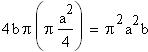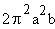Problem
Find the volume of the torus of radius a with inside radius b—in other words, the donut shape generated by rotating the circle x2 + (y – b)2 = a2 around the x axis (0 < a < b).
Solution
This is one of the tougher volume problems you'll come across. First, we'll try to visualize the shape.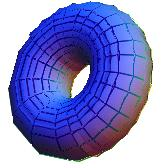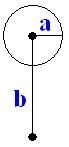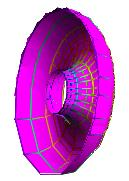There are lots of possible ways we could choose to integrate. Since this shape is symmetric around the y axis, we can integrate with respect to x over half of it and then double the resulting value. Here's the shell of half of the solid figure, sort of a half doughnut, showing only the "skin".
Each slice of this object is a washer of thickness Dx. So we move in the positive x direction, the outer radii decrease and inner radii increase: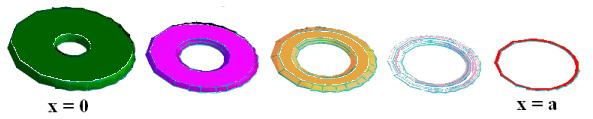The volume of the half torus is the sum of the areas of these washers from x = 0 to x = a, times the thickness Dx. The area of a washer is given by pro2pri2, where ro is the outer radius and ri is the inner radius. For a given value of a, we need to find formulas for ro and ri.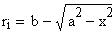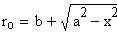The integral is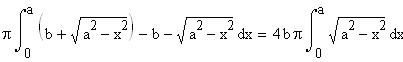The integral on the right hand side is the area of the quarter circle of radius a, or pa2/4. Therefore, the volume of half the torus is